• 数据分箱的重要性离散特征的增加和减少都很容易，易于模型的快速迭代；稀疏向量内积乘法运算速度快，计算结果方便存储，容易扩展；离散化后的特征对异常数据有很强的鲁棒性：比如一个特征是年龄>30是1，否则0。...
数据分箱的重要性离散特征的增加和减少都很容易，易于模型的快速迭代；稀疏向量内积乘法运算速度快，计算结果方便存储，容易扩展；离散化后的特征对异常数据有很强的鲁棒性：比如一个特征是年龄>30是1，否则0。如果特征没有离散化，一个异常数据“年龄300岁”会给模型造成很大的干扰；逻辑回归属于广义线性模型，表达能力受限；单变量离散化为N个后，每个变量有单独的权重，相当于为模型引入了非线性，能够提升模型表达能力，加大拟合；离散化后可以进行特征交叉，由M+N个变量变为M*N个变量，进一步引入非线性，提升表达能力；特征离散化后，模型会更稳定，比如如果对用户年龄离散化，20-30作为一个区间，不会因为一个用户年龄长了一岁就变成一个完全不同的人。当然处于区间相邻处的样本会刚好相反，所以怎么划分区间是门学问；特征离散化以后，起到了简化了逻辑回归模型的作用，降低了模型过拟合的风险。可以将缺失作为独立的一类带入模型。将所有变量变换到相似的尺度上。数据分箱的方法有：1.等距分箱def bin_frequency(x,y,n=10):total=y.count()bad=y.sum()good=total-badd1=pd.DataFrame({"x":x,"y":y,'bin':pd.qcut(x,n)})d2=d1.groupby('bin',as_index=True)d3=pd.DataFrame()d3['total']=d2.y.count()             ##每个箱中的总样本数d3['bad']=d2.y.sum()                 ##每个箱中的坏样本数d3['good']=d3['total']-d3['bad']     ##每个箱中的好样本数d3['bad_rate']=d3['bad']/d3['total']*100   ##每个箱中的坏样本率d3['%bad']=d3['bad']/bad*100               ##每个箱中的坏样本占总坏样本的比重d3['%good']=d3['good']/good*100            ##每个箱中的好样本占总好样本的比重d3['woe']=np.log(d3['%bad']/d3['%good'])iv=((d3['%bad']-d3['%good'])*d3['woe']).sum()d3.reset_index(inplace=True)print(d3,iv)2.等频分箱def bin_distance(x,y,n=10):   ##主要woe有可能为-inftotal=y.count()bad=y.sum()good=total-badd1=pd.DataFrame({"x":x,"y":y,'bin':pd.cut(x,n)})  ##等距分箱d2=d1.groupby('bin',as_index=True)d3=pd.DataFrame()d3['total']=d2.y.count()             ##每个箱中的总样本数d3['bad']=d2.y.sum()                 ##每个箱中的坏样本数d3['good']=d3['total']-d3['bad']     ##每个箱中的好样本数d3['bad_rate']=d3['bad']/d3['total']*100   ##每个箱中的坏样本率d3['%bad']=d3['bad']/bad*100               ##每个箱中的坏样本占总坏样本的比重d3['%good']=d3['good']/good*100            ##每个箱中的好样本占总好样本的比重d3['woe']=np.log(d3['%bad']/d3['%good'])iv=((d3['%bad']-d3['%good'])*d3['woe']).sum()d3.reset_index(inplace=True)print(d3,iv)3.自定义分箱def bin_self(x,y,cut):   ##cut:自定义分箱(list)total=y.count()bad=y.sum()good=total-badd1=pd.DataFrame({"x":x,"y":y,'bin':pd.cut(x,cut)})  ##等距分箱d2=d1.groupby('bin',as_index=True)d3=pd.DataFrame()d3['total']=d2.y.count()             ##每个箱中的总样本数d3['bad']=d2.y.sum()                 ##每个箱中的坏样本数d3['good']=d3['total']-d3['bad']     ##每个箱中的好样本数d3['bad_rate']=d3['bad']/d3['total']*100   ##每个箱中的坏样本率d3['%bad']=d3['bad']/bad*100               ##每个箱中的坏样本占总坏样本的比重d3['%good']=d3['good']/good*100            ##每个箱中的好样本占总好样本的比重d3['woe']=np.log(d3['%bad']/d3['%good'])iv=((d3['%bad']-d3['%good'])*d3['woe']).sum()d3.reset_index(inplace=True)print(d3,iv)bin_self(X['age'],Y,[-10,20,40,60,80,110])4.有监督分箱--决策树分箱先使用有监督学习决策树算法得到决策树的叶子节点，把叶子节点的值作为分箱的边界，再使用自定义分箱得到分箱结果。from sklearn.tree import DecisionTreeClassifierdef decision_tree_bin(x,y):'''利用决策树获得最优分箱的边界值列表'''boundary = []  # 待return的分箱边界值列表x = x.fillna(-999).values  # 填充缺失值y = y.valuesclf = DecisionTreeClassifier(criterion='entropy',    #“信息熵”最小化准则划分max_leaf_nodes=6,       # 最大叶子节点数min_samples_leaf=0.05)  # 叶子节点样本数量最小占比clf.fit(x.reshape(-1, 1), y)  # 训练决策树n_nodes = clf.tree_.node_countchildren_left = clf.tree_.children_leftchildren_right = clf.tree_.children_rightthreshold = clf.tree_.thresholdfor i in range(n_nodes):if children_left[i] != children_right[i]:  # 获得决策树节点上的划分边界值boundary.append(threshold[i])boundary.sort()min_x = x.min()max_x = x.max() + 0.1  # +0.1是为了考虑后续groupby操作时，能包含特征最大值的样本boundary = [min_x] + boundary + [max_x]return boundaryboundary_list=decision_tree_bin(X['age'],Y)bin_self(X['age'],Y,boundary_list)5.无监督分箱--卡方分箱卡方分箱是再实际工作中常用的分箱方法，这里我先介绍一下卡方检验，卡方检验是检验两个分类变量之间的关联关系，其根本思想就是在于比较理论频数和实际频数的吻合程度或拟合优度问题。卡方检验的H0：观察频数与期望频数没有差别。然后计算卡方值：计算公式为：其中A为实际值，T为理论值，如果卡方值越大，越能拒绝原假设。卡方分箱采取自底向上不断合并的方法完成分箱操作。在每一步的合并过程中，依靠最小的卡方值来寻找最优的合并项。其核心思想是，如果某两个区间可以被合并，那么这两个区间的坏样本需要有最接近的分布，进而意味着两个区间的卡方值是最小的。于是ChiMerge的步骤如下：将数值变量A排序后分成区间较多的若干组，设为A_1,A_2,…,A_n计算A_1 与A_2合并后的卡方值，A_2 与A_3合并后的卡方值，直至A_(n-1) 与A_n合并后的卡方值找出上一步所有合并后的卡方值中最小的一个，假设为A_(i-1) 与A_i,将其合并形成新的A_(i-1)不断重复2和3，直至满足终止条件import mathimport pandas as pdimport numpy as np# 先用卡方分箱输出变量的分割点def split_data(df,col,split_num):"""df: 原始数据集col:需要分箱的变量split_num:分割点的数量"""df2 = df.copy()count = df2.shape # 总样本数n = math.floor(count/split_num) # 按照分割点数目等分后每组的样本数split_index = [i*n for i in range(1,split_num)] # 分割点的索引values = sorted(list(df2[col])) # 对变量的值从小到大进行排序split_value = [values[i] for i in split_index] # 分割点对应的valuesplit_value = sorted(list(set(split_value))) # 分割点的value去重排序return split_valuedef assign_group(x,split_bin):"""x:变量的valuesplit_bin:split_data得出的分割点list"""n = len(split_bin)if x<=min(split_bin):return min(split_bin) # 如果x小于分割点的最小值，则x映射为分割点的最小值elif x>max(split_bin): # 如果x大于分割点的最大值，则x映射为分割点的最大值return 10e10else:for i in range(n-1):if split_bin[i]return split_bin[i+1]def bin_bad_rate(df,col,target,grantRateIndicator=0):"""df:原始数据集col:原始变量/变量映射后的字段target:目标变量的字段grantRateIndicator:是否输出总体的违约率"""total = df.groupby([col])[target].count()bad = df.groupby([col])[target].sum()total_df = pd.DataFrame({'total':total})bad_df = pd.DataFrame({'bad':bad})regroup = pd.merge(total_df,bad_df,left_index=True,right_index=True,how='left')regroup = regroup.reset_index()regroup['bad_rate'] = regroup['bad']/regroup['total']  # 计算根据col分组后每组的违约率dict_bad = dict(zip(regroup[col],regroup['bad_rate'])) # 转为字典形式if grantRateIndicator==0:return (dict_bad,regroup)total_all= df.shapebad_all = df[target].sum()all_bad_rate = bad_all/total_all # 计算总体的违约率return (dict_bad,regroup,all_bad_rate)def cal_chi2(df,all_bad_rate):"""df:bin_bad_rate得出的regroupall_bad_rate:bin_bad_rate得出的总体违约率"""df2 = df.copy()df2['expected'] = df2['total']*all_bad_rate # 计算每组的坏用户期望数量combined = zip(df2['expected'],df2['bad']) # 遍历每组的坏用户期望数量和实际数量chi = [(i-i)**2/i for i in combined] # 计算每组的卡方值chi2 = sum(chi) # 计算总的卡方值return chi2def assign_bin(x,cutoffpoints):"""x:变量的valuecutoffpoints:分箱的切割点"""bin_num = len(cutoffpoints)+1 # 箱体个数if x<=cutoffpoints:  # 如果x小于最小的cutoff点，则映射为Bin 0return 'Bin 0'elif x>cutoffpoints[-1]: # 如果x大于最大的cutoff点，则映射为Bin(bin_num-1)return 'Bin{}'.format(bin_num-1)else:for i in range(0,bin_num-1):if cutoffpoints[i]return 'Bin{}'.format(i+1)def ChiMerge(df,col,target,max_bin=5,min_binpct=0):col_unique = sorted(list(set(df[col]))) # 变量的唯一值并排序n = len(col_unique) # 变量唯一值得个数df2 = df.copy()if n>100:  # 如果变量的唯一值数目超过100，则将通过split_data和assign_group将x映射为split对应的valuesplit_col = split_data(df2,col,100)  # 通过这个目的将变量的唯一值数目人为设定为100df2['col_map'] = df2[col].map(lambda x:assign_group(x,split_col))else:df2['col_map'] = df2[col]  # 变量的唯一值数目没有超过100，则不用做映射# 生成dict_bad,regroup,all_bad_rate的元组(dict_bad,regroup,all_bad_rate) = bin_bad_rate(df2,'col_map',target,grantRateIndicator=1)col_map_unique = sorted(list(set(df2['col_map'])))  # 对变量映射后的value进行去重排序group_interval = [[i] for i in col_map_unique]  # 对col_map_unique中每个值创建list并存储在group_interval中while (len(group_interval)>max_bin): # 当group_interval的长度大于max_bin时，执行while循环chi_list=[]for i in range(len(group_interval)-1):temp_group = group_interval[i]+group_interval[i+1] # temp_group 为生成的区间,list形式，例如[1,3]chi_df = regroup[regroup['col_map'].isin(temp_group)]chi_value = cal_chi2(chi_df,all_bad_rate) # 计算每一对相邻区间的卡方值chi_list.append(chi_value)best_combined = chi_list.index(min(chi_list)) # 最小的卡方值的索引# 将卡方值最小的一对区间进行合并group_interval[best_combined] = group_interval[best_combined]+group_interval[best_combined+1]# 删除合并前的右区间group_interval.remove(group_interval[best_combined+1])# 对合并后每个区间进行排序group_interval = [sorted(i) for i in group_interval]# cutoff点为每个区间的最大值cutoffpoints = [max(i) for i in group_interval[:-1]]# 检查是否有箱只有好样本或者只有坏样本df2['col_map_bin'] = df2['col_map'].apply(lambda x:assign_bin(x,cutoffpoints)) # 将col_map映射为对应的区间Bin# 计算每个区间的违约率(dict_bad,regroup) = bin_bad_rate(df2,'col_map_bin',target)# 计算最小和最大的违约率[min_bad_rate,max_bad_rate] = [min(dict_bad.values()),max(dict_bad.values())]# 当最小的违约率等于0，说明区间内只有好样本，当最大的违约率等于1，说明区间内只有坏样本while min_bad_rate==0 or max_bad_rate==1:bad01_index = regroup[regroup['bad_rate'].isin([0,1])].col_map_bin.tolist()# 违约率为1或0的区间bad01_bin = bad01_indexif bad01_bin==max(regroup.col_map_bin):cutoffpoints = cutoffpoints[:-1] # 当bad01_bin是最大的区间时，删除最大的cutoff点elif bad01_bin==min(regroup.col_map_bin):cutoffpoints = cutoffpoints[1:] # 当bad01_bin是最小的区间时，删除最小的cutoff点else:bad01_bin_index = list(regroup.col_map_bin).index(bad01_bin) # 找出bad01_bin的索引prev_bin = list(regroup.col_map_bin)[bad01_bin_index-1] # bad01_bin前一个区间df3 = df2[df2.col_map_bin.isin([prev_bin,bad01_bin])](dict_bad,regroup1) = bin_bad_rate(df3,'col_map_bin',target)chi1 = cal_chi2(regroup1,all_bad_rate)  # 计算前一个区间和bad01_bin的卡方值later_bin = list(regroup.col_map_bin)[bad01_bin_index+1] # bin01_bin的后一个区间df4 = df2[df2.col_map_bin.isin([later_bin,bad01_bin])](dict_bad,regroup2) = bin_bad_rate(df4,'col_map_bin',target)chi2 = cal_chi2(regroup2,all_bad_rate) # 计算后一个区间和bad01_bin的卡方值if chi1cutoffpoints.remove(cutoffpoints[bad01_bin_index-1])else:  # 当chi1>=chi2时,删除bin01对应的cutoff点cutoffpoints.remove(cutoffpoints[bad01_bin_index])df2['col_map_bin'] = df2['col_map'].apply(lambda x:assign_bin(x,cutoffpoints))(dict_bad,regroup) = bin_bad_rate(df2,'col_map_bin',target)# 重新将col_map映射至区间，并计算最小和最大的违约率，直达不再出现违约率为0或1的情况，循环停止[min_bad_rate,max_bad_rate] = [min(dict_bad.values()),max(dict_bad.values())]# 检查分箱后的最小占比if min_binpct>0:group_values = df2['col_map'].apply(lambda x:assign_bin(x,cutoffpoints))df2['col_map_bin'] = group_values # 将col_map映射为对应的区间Bingroup_df = group_values.value_counts().to_frame()group_df['bin_pct'] = group_df['col_map']/n # 计算每个区间的占比min_pct = group_df.bin_pct.min() # 得出最小的区间占比while min_pct2: # 当最小的区间占比小于min_pct且cutoff点的个数大于2，执行循环# 下面的逻辑基本与“检验是否有箱体只有好/坏样本”的一致min_pct_index = group_df[group_df.bin_pct==min_pct].index.tolist()min_pct_bin = min_pct_indexif min_pct_bin == max(group_df.index):cutoffpoints=cutoffpoints[:-1]elif min_pct_bin == min(group_df.index):cutoffpoints=cutoffpoints[1:]else:minpct_bin_index = list(group_df.index).index(min_pct_bin)prev_pct_bin = list(group_df.index)[minpct_bin_index-1]df5 = df2[df2['col_map_bin'].isin([min_pct_bin,prev_pct_bin])](dict_bad,regroup3) = bin_bad_rate(df5,'col_map_bin',target)chi3 = cal_chi2(regroup3,all_bad_rate)later_pct_bin = list(group_df.index)[minpct_bin_index+1]df6 = df2[df2['col_map_bin'].isin([min_pct_bin,later_pct_bin])](dict_bad,regroup4) = bin_bad_rate(df6,'col_map_bin',target)chi4 = cal_chi2(regroup4,all_bad_rate)if chi3cutoffpoints.remove(cutoffpoints[minpct_bin_index-1])else:cutoffpoints.remove(cutoffpoints[minpct_bin_index])return cutoffpointscut=chisqbin.ChiMerge(data,'age','SeriousDlqin2yrs',max_bin=5,min_binpct=0)def chisqbin_bin(x,y,cutpoints):inf = float('inf')ninf = float('-inf')total=y.count()bad=y.sum()good=total-badcutpoints.insert(0,ninf)cutpoints.append(inf)d1=pd.DataFrame({"x":x,"y":y,'bin':pd.cut(x,cut)})  ##等距分箱d2=d1.groupby('bin',as_index=True)d3=pd.DataFrame()d3['total']=d2.y.count()             ##每个箱中的总样本数d3['bad']=d2.y.sum()                 ##每个箱中的坏样本数d3['good']=d3['total']-d3['bad']     ##每个箱中的好样本数d3['bad_rate']=d3['bad']/d3['total']*100   ##每个箱中的坏样本率d3['%bad']=d3['bad']/bad*100               ##每个箱中的坏样本占总坏样本的比重d3['%good']=d3['good']/good*100            ##每个箱中的好样本占总好样本的比重d3['woe']=np.log(d3['%bad']/d3['%good'])iv=((d3['%bad']-d3['%good'])*d3['woe']).sum()print(d3,iv)return d3,ivchisqbin_bin(X['age'],Y,cut)
展开全文• 要对一个特征进行WOE编码，需要首先把这个变量进行分组处理（也叫离散化、分箱等等，说的都是一个意思）。分组后，对于第i组，WOE的计算公式如下： WOE = ln(pyi/pni) = ln((yi/yt)/(ni/nt)) = ln((yi/ni)/(yt/nt)) ...
IV的概念和作用
概念：
IV的全称是Information Value，中文意思是信息价值，或者信息量。
作用：
我们构建分类模型时，经常需要对特征进行筛选。比如我们有200个候选特征，通常情况下，不会直接把200个特征直接放到模型中去进行训练，
挑选特征的过程是个比较复杂的过程，需要考虑的因素很多，比如：特征的预测能力，特征之间的相关性，特征在业务上的可解释性等等。但是，其中最主要和最直接的衡量标准是特征的预测能力。我们需要一些具体的量化指标来衡量每自变量的预测能力。IV就是这样一种指标，他可以用来衡量自变量的预测能力。
什么是WOE
WOE的全称是“Weight of Evidence”，即证据权重。WOE是对原始特征的一种编码形式。要对一个特征进行WOE编码，需要首先把这个变量进行分组处理（也叫离散化、分箱等等，说的都是一个意思）。分组后，对于第i组，WOE的计算公式如下：
WOE = ln(pyi/pni) = ln((yi/yt)/(ni/nt)) = ln((yi/ni)/(yt/nt))
其中，pyi是这个组中正例样本占整个样本中正例样本的比例，pni是这个组中负例样本占整个样本中负例样本的比例，#yi是这个组中正例样本的数量，#ni是这个组中负例样本的数量，#yT是整个样本中所有正例样本的数量，#nT是整个样本中所有负例样本的数量。
IV的计算公式
IV = (pyi - pni)*WOE = (pyi - pni) * ln((yi/yt)/(ni/nt)) = ln((yi/ni)/(yt/nt))
有了一个特征各分组的IV值，我们就可以计算整个特征的IV值，方法很简单，就是把各分组的IV相加：其中n就是分组的个数
关于IV和WOE的进一步思考：为什么用IV而不是直接用WOE？？？
第一个原因，当我们衡量一个特征的预测能力时，我们所使用的指标值不应该是负数，否则，说一个特征的预测能力的指标是-2.3，听起来很别扭。从这个角度讲，乘以pyn这个系数，保证了特征每个分组的结果都是非负数，你可以验证一下，当一个分组的WOE是正数时，pyn也是正数，当一个分组的WOE是负数时，pyn也是负数，而当一个分组的WOE=0时，pyn也是0。
当然，上面的原因不是最主要的，因为其实我们上面提到的绝对值这个指标也可以完全避免负数的出现。
更主要的原因，也就是第二个原因是，乘以pyn后，体现出了特征当前分组中个体的数量占整体个体数量的比例（样本占整体样本的比例），对变量预测能力的影响。怎么理解这句话呢？我们还是举个例子。
分箱
数据分箱（也称为离散分箱或分段）是一种数据预处理技术，用于减少次要观察误差的影响，是一种将多个连续值分组为较少数量的“分箱”的方法。说白了就是将连续型特征进行离散化。
注意：
分箱的数据不一定必须是数字，它们可以是任何类型的值，如“狗”，“猫”，“仓鼠”等。 分箱也用于图像处理，通过将相邻像素组合成单个像素，它可用于减少数据量
分箱的作用&意义
离散特征的增加和减少都很容易，易于模型的快速迭代，提升计算速度
特征离散化后，模型会更稳定，比如如果对用户年龄离散化，20-30作为一个区间，不会因为一个用户年龄长了一岁就变成一个完全不同的人。
特征离散化以后，起到了简化了模型的作用，可以适当降低了模型过拟合的风险。
pandas实现数据的分箱：pd.cut()
import numpy as np
import pandas as pd
from pandas import Series,DataFrame
score_list = np.linspace(0,20,num=11)
print(score_list)
bins = [0,4,8,16,20]
score_category = pd.cut(score_list,bins)
# score_category
print(pd.value_counts(score_category))

bins = 3
score_category = pd.cut(score_list,bins,labels=['a','b','c'])
print(pd.value_counts(score_category))
print(score_category.tolist())

import sklearn.datasets as datasets
feature = bc.data
target = bc.target
feature[:,1].max(),feature[:,1].min()
feature_bins = pd.cut(feature[:,1],bins=5)
feature_bins.value_counts()
gi = pd.crosstab(feature_bins,target)
gb = pd.Series(data=target).value_counts()
gbi = (gi/gi)/(gb/gb)
woe = np.log(gbi)
dic = woe.to_dict()
woe_bins = feature_bins.map(dic)
woe_bins
woe_bins.tolist()


什么是样本类别分布不均衡？
举例说明，在一组样本中不同类别的样本量差异非常大，比如拥有1000条数据样本的数据集中，有一类样本的分类只占有10条，此时属于严重的数据样本分布不均衡
样本类别分布不均衡导致的危害？
样本类别不均衡将导致样本量少的分类所包含的特征过少，并很难从中提取规律；即使得到分类模型，也容易产生过度依赖与有限的数据样本而导致过拟合问题，当模型应用到新的数据上时，模型的准确性会很差。
解决方法：
通过过抽样和欠抽样解决样本不均衡
也可以叫做上采样，和下采样
过抽样（over-sampling）：
from imblearn.over_sampling import SMOTE
通过增加分类中少数类样本的数量来实现样本均衡，比较好的方法有SMOTE算法。
SMOTE算法原理介绍：
简单来说smote算法的思想是合成新的少数类样本，合成的策略是对每个少数类样本a，从它的最近邻中随机选一个样本b，然后在a、b之间的连线上随机选一点作为新合成的少数类样本。
参数：k_neighbors
找出类别少的样本点周围最近的k个邻居
import pandas as pd
import numpy as np
x = np.random.randint(0,100,size=(100,3))
y = pd.Series(data=np.random.randint(0,1,size=(95,)))
y = y.append(pd.Series(data=[1,1,1,1,1]),ignore_index=False).values
y = y.reshape(-1,1)
all_data_np = np.concatenate((x,y),axis=1)
np.random.shuffle(all_data_np)
df = pd.DataFrame(all_data_np)

df.value_counts()
x = df[[0,1,2]]
y = df
from imblearn.over_sampling import SMOTE
s = SMOTE(k_neighbors=3)
feature, target = s.fit_sample(x,y)




展开全文python
• 评分卡介绍评分卡的种类——ABC卡FICO信用分1、FICO信用分简介2、FICO信用分的评判因素数据准备1、需要排除异常行为2、解释指标的选择3、目标变量的确立变量分箱——WOE转换评分的计算与分值分配模型的评价——模型...
评分卡介绍评分卡的种类——ABC卡FICO信用分1、FICO信用分简介2、FICO信用分的评判因素数据准备1、需要排除异常行为2、解释指标的选择3、目标变量的确立变量分箱——WOE转换评分的计算与分值分配模型的评价——模型区分度1、KS值2、GINI指数
评分卡的种类——ABC卡

A卡（Application score card）申请评分卡，贷前，一般可做贷款0-1年的信用分析

B卡（Behavior score card）行为评分卡， 贷中，在申请人有了一定行为后，有了较大数据进行的分析，一般为3-5年

C卡（Collection score card）催收评分卡，贷后，需加入催收后客户反应等属性数据

FICO信用分
1、FICO信用分简介
FICO信用分是最常用的一种普通信用分。由于美国三大信用局都使用FICO信用分，每一份信用报告上都附有FICO信用分，以致FICO信用分成为信用分的代名词。该分数是一个[300,850]区间的分数段。
信用评分达到680分以上，贷款方就可以认为借款人的信用卓著，可以毫不迟疑地同意发放款；信用评分低于620分，贷款方或者要求借款人增加担保，或者干脆寻找各种理由拒绝贷款；如果借款人的信用评分介于620-680分之间，贷款方就要作进一步的调查核实，采用其它的信用分析工具，作个案处理。
2、FICO信用分的评判因素
部分评判标准和影响因素（变量）：
（1）信用偿还历史（占总影响因素的35%）：
各种信用账户的还款记录（信用卡、零售账户 、分期偿还贷款、金融公司账户、抵押贷款）、公开记录及支票存款记录（破产记录、丧失抵押品赎回权记录、法律诉讼事件、留置权记录及判决）、逾期偿还的具体情况（逾期的天数、未偿还的金额、逾期还款的次数和逾期发生时距现在的时间长度等）
（2）信用账户数（占总影响因素的30%）：
分析对于一个客户, 究竟多少个信用账户是足够多的, 从而能够准确反应出客户的还款能力
（3）信用账龄（占总因素的15%）：
该用户信用历史越长，FICO信用分越高。该项因素主要指信用账户的账龄,也包括新开立的信用账户的账龄, 以及平均信用账户账龄。
（4）新开立的账户（占总因素的10%）：
在很短时间内开立多个信用账户的客户具有更高的信用风险, 尤其是那些信用历史不长的人。该类因素包括：新开立的信用账户数、新开立的信用账户账龄、目前的信用申请数量。
（5）在使用的信用类型（占总因素的10%）：
主要分析客户的信用卡账户、零售账户、分期付款账户、金融公司账户和抵押贷款账户的混合使用情况, 具体包括: 持有的信用账户类型和每种类型的信用账户数。
数据准备
1、需要排除异常行为
销户、按条例拒绝、特殊账户（卡丢失、死亡、未成年、出国、员工账户、VIP账户）；
2、解释指标的选择
（1）申请评分所需指标：参考上述FICO信用分的	评判标准
（2）行为评分所需指标：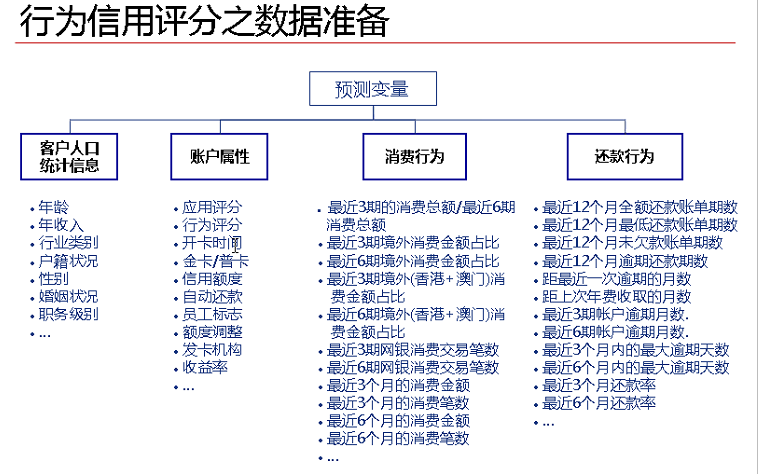3、目标变量的确立
目标变量为坏客户/好客户
坏客户：观察窗口内，逾期60/90/120天的用户
因此在此之前还需要确定观察窗口和违约时间的长短。
变量分箱——WOE转换
WOE为优势比，将各个连续变量细分为若干组后，每组的WOE为：
$WOE = ln \frac{该组好客户占比}{该组坏客户占比}*100\%$
*注意：”该组好客户占比“指的是该组好用户数/所有的好用户数
1、如果计算得到的WOE与违约概率具有某种线性关系，或者WOE与目标变量之间的有U型或者倒U型的非线性关系，说明该种分组方式合理，否则需要调整分组方式，如果无论怎么分组均无法达到上述两种情况，说明该变量对目标变量的解释性较弱。
2、模型拟合出来的自变量系数应该都是正数，如果结果中出现了负数，应当考虑是否是来自自变量多重共线性的影响——可以计算各个变量的VIF值，删去那些具有明显多重共线性的变量。
3、WOE编码之后，自变量其实具备了某种标准化的性质，也就是说，自变量内部的各个取值之间都可以直接进行比较（WOE之间的比较），而不同自变量之间的各种取值也可以通过WOE进行直接的比较。
4、如果遇到分箱后某组的坏客户数量为0，则需要合并分箱，保证每个分箱中必须同时包含好用户和坏用户
此外，IV值(information value)衡量的是某一个变量的信息量，可用来表示一个变量的预测能力。公式如下(假设该变量经过变量分箱后被分为m组)：
$IV = \sum^{m}_{i = 1}(好用户占比-坏用户占比)*WOE_{i}$
不同IV值表示的变量的预测能力大小如下表：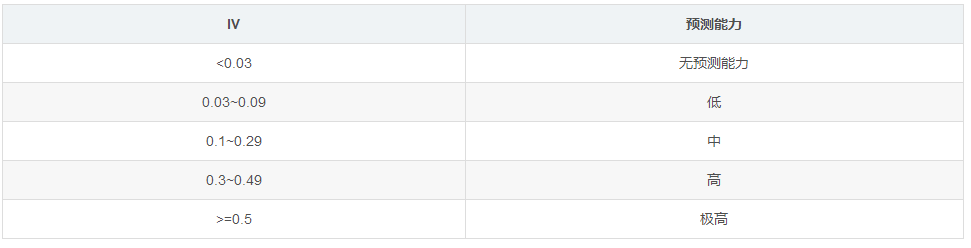举个例子：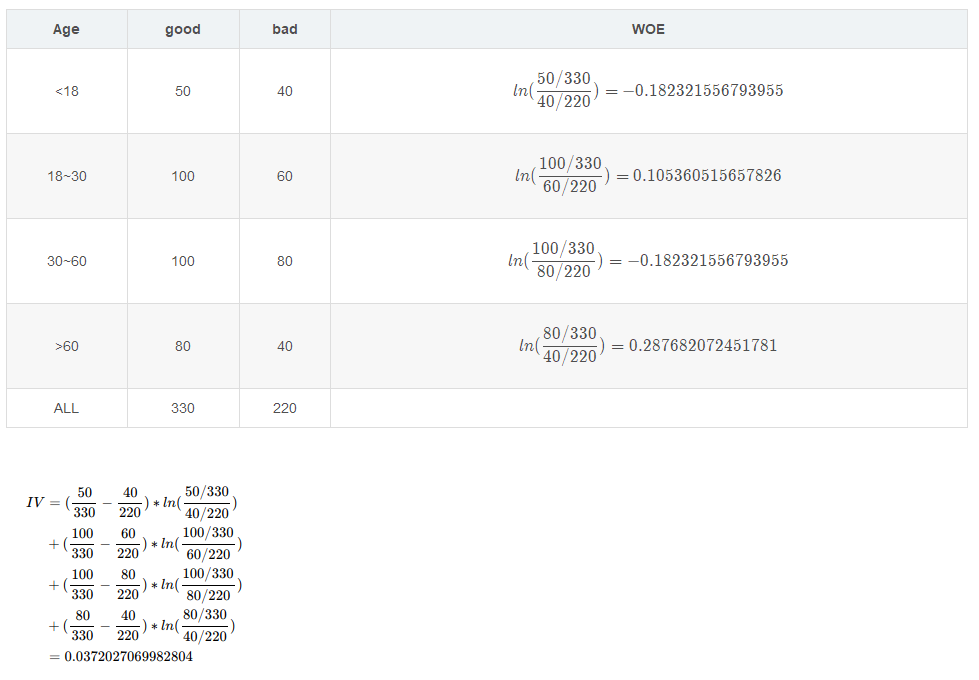此例中IV值较小，预测能力低，建议重新调整分箱。
评分的计算与分值分配
首先定义$odds = \frac{好客户占比}{坏客户占比} = \frac{p}{1-p}$
总分 $score_{总} = a + b*ln(odds)$
接下来需要求解a，b的值，假设当odds=$\theta_0$时，得分为$p_0$，假设刻度为$\delta_p$，那么当odds=$2\theta_0$时，得分应为$p_0$+$\delta_p$，由此可以列出下式：
$p_0 = a + b*ln(\theta_0)$
$p_0+\delta_p =a + b*ln(2\theta_0)$
其中，$p_0$和$\delta_p$可以人为设定，联立上面两式求得a，b的值，带入score公式，得到不同$\theta_0$下的评分卡的分值——此处的$\theta_0$就是odds，也就是逻辑回归模型预测得到的结果$p$。至此，各个样本的计算结果已经得到。
模型的评价——模型区分度
1、KS值
KS的计算步骤如下：

计算每个评分区间的好坏用户数。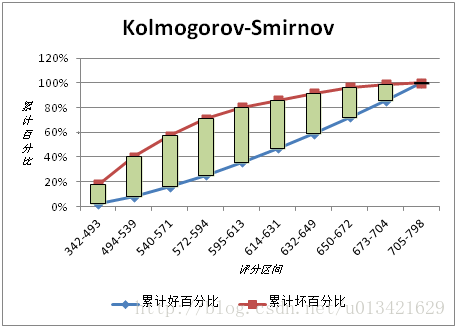KS用于模型风险区分能力进行评估，好坏样本累计差异越大，KS指标越大，那么模型的风险区分能力越强。

2、GINI指数
GINI系数的计算步骤如下：

计算每个评分区间的好坏账户数。
计算出图中阴影部分面积，阴影面积占直角三角形ABC面积的百分比，即为GINI系数。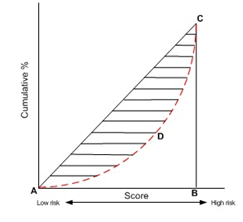【注】本文引用了以下文章：
https://blog.csdn.net/sinat_26917383/article/details/51721107
https://blog.csdn.net/sscc_learning/article/details/78591210
https://blog.csdn.net/u013421629/article/details/78217498


展开全文评分卡模型 GINI
• 1 IV编码与WOE编码 1.1 IV编码 1.1.1 介绍 IV编码，Information Value，指的是信息价值或者信息量。 IV编码可以理解为特征筛选的量化指标， 用于衡量数据特征的预测能力或者在模型预测过程中对预测结果的影响程度。 ...
机器学习
1 IV与WOE
1.1 IV
IV，Information Value，指的是信息价值或者信息量。
IV可以理解为特征筛选的量化指标， 用于衡量数据特征的预测能力或者在模型预测过程中对预测结果的影响程度。
关于衡量特征预测能力的理解
假设在一个分类问题中，数据集分为两种标签类别：Y1和Y2。对于一个待预测的样本A，要判断其属于类别Y1还是类别Y2，我们需要一定的信息，假设这个信息总量是I，而这些信息蕴含在待预测样本的所有特征C1，C2，…，Cn中。对于某个特征Ci，如果特征Ci包含的信息越多，那么它对于判断样本A的类别这个问题的贡献就越大，即特征Ci的信息价值就越大，等价于特征Ci的IV就越大，因此特征Ci就越应该优先选择出来并用于训练模型。
1.2 WOE介绍及计算公式
WOE，Weight of Evidence，指的是证据权重，WOE是对原始特征的一种编码形式。要对一个特征进行WOE编码，首先需要对这个变量进行分组处理，分组处理也称为离散化、分箱等。
WOE的计算公式
对于分组后的第i组
$WOE_i = ln({{P_{y_i}} \over {P_{n_i}}}) = ln({{\# y_i / \# y_t} \over {\# n_i / \# n_t}})$
$P_{y_i}$表示组中正例样本数量占全部正例样本数量的比例；
$P_{n_i}$表示组中负例样本数量占全部负例样本数量的比例；
$\# y_i$表示组中正例样本数量，$\# y_t$表示全部正例样本数量；
$\# n_i$表示组中负例样本数量，$\# n_t$表示全部负例样本数量。
从公式中可以看出，WOE实际上表示
“指定组中正例样本数量占样本集中所有正例样本数量的比例”与“当前组中负例样本数量占样本集中所有负例样本数量的比例”之间的差异。
对于分组后的第i组，
$WOE_i = ln({{\# y_i / \# y_t} \over {\# n_i / \# n_t}}) = ln({{\# y_i / \# n_i} \over {\# y_t / \# n_t}})$

对于给定的样本集，${\# y_t / \# n_t}$ 是固定值，因此WOE也可以反映出指定组中正例样本数量与负例样本数量的比值。WOE越大，这个组的正例样本占比就越大。
WOE值可正可负，每一组的WOE值的正负由当前组中正例样本数量与负例样本数量的比例，与样本整体中正例样本数量与负例样本数量的比例的大小关系决定。
如果某一组的WOE值为正，与整体数据相比这个组更偏向于将样本划分到正例样本中，反之，如果某一组的WOE值为负，与整体数据相比这个组更偏向于将样本划分到负例样本中。
如果某一组的WOE值为0，则说明这个组中正负例样本数量的比例与整体情况相同。

1.3 IV计算公式
IV的计算公式是以WOE的计算公式为基础的。
对于分组后的第i组
\begin{aligned} IV_i =& (P_{y_i} - P_{n_i}) \times WOE_i \\ IV_i =& (P_{y_i} - P_{n_i}) \times ln({{P_{y_i}} \over {P_{n_i}}}) \\ IV_i =& ({\# y_i / \# y_t} - {\# n_i / \# n_t}) \times ln({{\# y_i / \# y_t} \over {\# n_i / \# n_t}}) \end{aligned}

从IV的计算公式可以看出，如果某一组的正例样本数量与负例样本数量的比例，与整体数据的正例样本数量与负例样本数量的比例相差越大，则这个组的IV值就越大。
如果某一组的正例样本数量与负例样本数量的比例，与整体数据的正例样本数量与负例样本数量的比例相等，则IV值为0。
IV值不可能为负数。

1.4 案例
1.4.1 案例介绍
某公司准备举行一个活动，举行活动前在小范围的客户群体中进行一次试点，收集一些用户对这次活动响应，然后希望利用这些数据构造模型，并预测假如举行这次的活动，是否能够得到很好的响应。
假设从公司的客户列表中随机抽取了100000名客户进行了营销活动测试，其中响应的客户有10000个，并且已经获取了这些响应客户的一些变量(特征)，作为模型的候选变量集，包括:

最近一个月是否存在购买行为；
最近一次购买的金额；
最近一笔购买的商品类别；
是否是公司的VIP客户。

假设已经对这些变量进行了离散化处理，统计结果如下所示。
(1) 最近一个月是否存在购买行为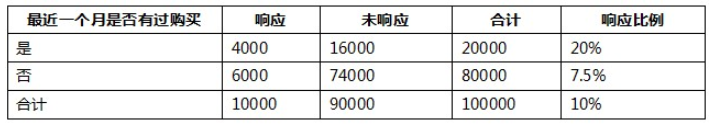(2) 最近一次购买的金额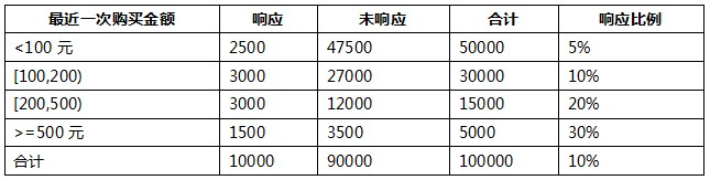(3) 最近一笔购买的商品类别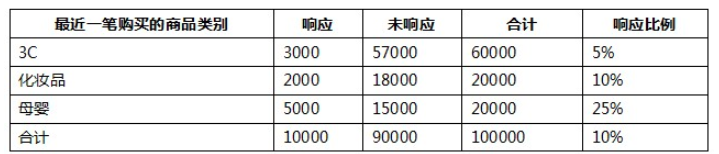(4) 是否是公司的VIP客户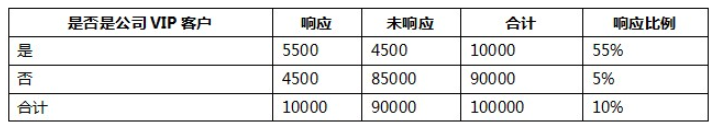1.4.2 计算WOE和IV
以最近一次的购买金额为例，将响应特征设为正例，未响应特征设为反例。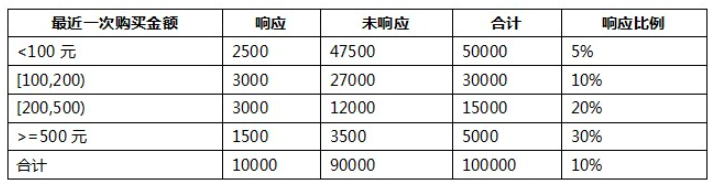1.4.2.1 计算WOE
计算特征(最近一次的购买金额)的WOE。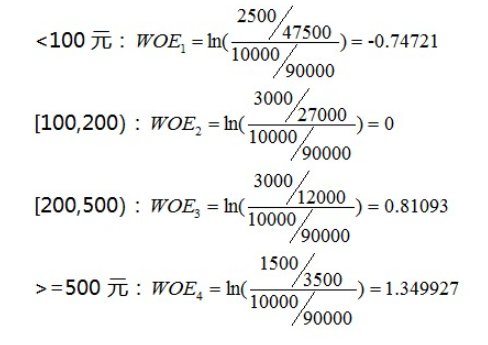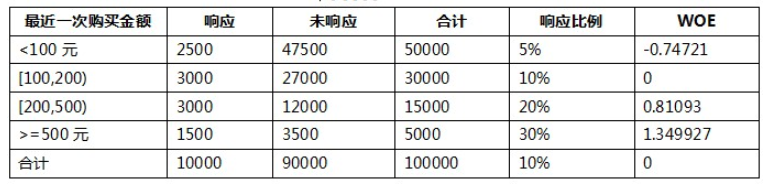将响应特征设为正例，未响应特征设为反例。

由上面的数据可以看出，WOE值反映了每个组内响应数据数量与未响应数据数量的占比情况。对于所有的分组，响应的比例越大，WOE值越大。
当某一组的WOE值为正时，与整体相比这个组的数据更偏向于响应，反之如果某一组的WOE值为负，与整体相比这个组的数据更偏向于不响应。
如果某一组的WOE值为0，则说明这个组的特征偏向与整体数据相同。

1.4.2.2 计算IV
计算特征(最近一次的购买金额)的IV。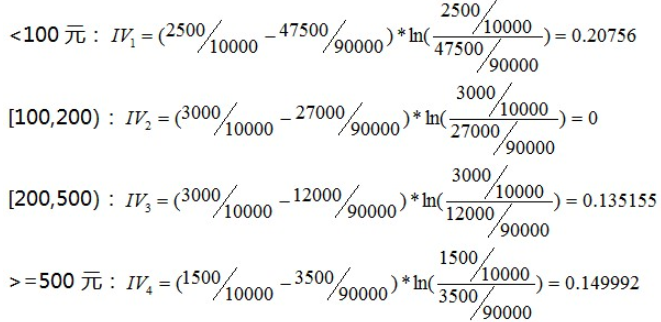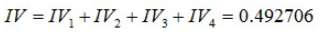1.4.3 比较IV，进行特征选择

特征
IV

最近一个月是否存在购买行为
0.250225

最近一次购买的金额
0.492706

最近一笔购买的商品类别
0.615276

是否是公司的VIP客户
1.565504

通过比较各个特征的IV值，发现特征(是否是公司的VIP客户)的IV值最高，说明这个特征的预测能力最强。
如果需要选择出某些特征去训练模型，则可以选择IV值较高的特征。
1.4.4 思考
进行特征选择时，为什么使用IV而不是直接使用WOE作为选择标准。
每个特征的IV和WOE都表示当前分组对这个特征的预测能力。

从公式可以看出，IV值不能为负值，而WOE值可正可负。
如果使用WOE作为特征选择的标准，不能直接相加，而是计算WOE的绝对值的和，增加了计算成本。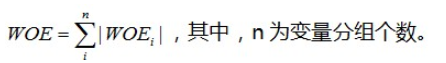特征选择时也需要考虑分组后每一组的样本数量占比。

例如，基于某个特征A进行分组，A特征只有两个：0和1。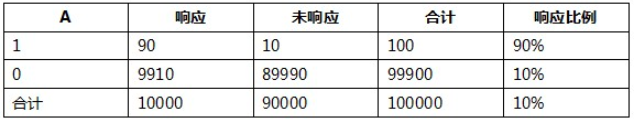当特征A取1时，响应比例达到了90%，但是与另一组(A=0)相比，这个组(A=1)的样本数量在整体样本中的占比非常小，因此仅使用响应比例不能很好的进行特征选择。
与WOE相比，IV的计算公式是在WOE的基础上增加了系数($P_{y_i} - P_{n_i}$)，这个系数中蕴含了这个分组样本在整体样本中的数量占比情况。因此，进行特征选择时，使用IV作为标准可以考虑到每个分组的样本数量的占比情况，更加严谨。
2 分箱
2.1 介绍
数据分箱，也称为离散分箱或者分段，是一种数据预处理技术，用于将连续型特征进行离散化处理，可以将多个连续值按照取值范围分别装入有限数量的箱子中，通过分箱操作可以减少次要观察误差的影响。
例如，有一组人员年龄统计数据。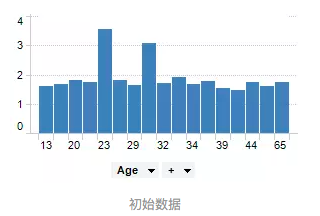通过分箱操作，可以将每个箱子的范围扩大，将箱子的数量减少。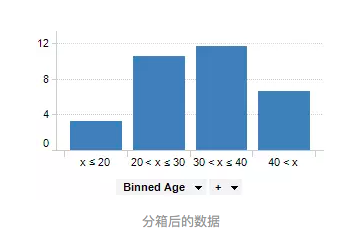2.2 分箱的作用

分箱后易于增加或减少离散特征，易于模型的快速迭代，提升计算速度；
特征离散化后，模型会更稳定；
例如对用户年龄进行离散化处理后，以10年作为一个区间长度，当时间过了一年，不处于年龄区间边界的用户不会因为年龄长一岁就移动至其它区间中；
特征离散化起到了简化模型的作用，可以适当降低了模型过拟合的风险。

2.3 代码实现
2.3.1 cut
cut根据样本数据的数值来确定箱子的均匀间隔，即每个箱子的间距尽量相等。
pd.cut(x, bins, right=True, labels=None, retbins=False, precision=3, include_lowest=False, duplicates='raise')

x - 待分箱的一维数据；
bins - 指定分隔区间；
若输入int数字则表示分箱后箱子数量；
若输入list或np.array则表示指定了每个箱子的范围，区间范围默认是左开右闭。

right - 布尔值，默认为True，表示区间范围是否包含右侧；
labels - 为分箱后的箱子设置标签，数量必须和箱子数量一致。

import numpy as np
import pandas as pd
from pandas import Series, DataFrame

score_list = np.linspace(0, 20, num=11)
print(score_list)
# [ 0.  2.  4.  6.  8. 10. 12. 14. 16. 18. 20.]


通过参数bins指定箱子数量。

bins = 3
score_cat = pd.cut(score_list, bins)
print(pd.value_counts(score_cat))
'''
(13.333, 20.0]     4
(-0.02, 6.667]     4
(6.667, 13.333]    3
'''


通过参数bins指定箱子范围。

bins=[0, 4, 8, 16, 20]
score_cat = pd.cut(score_list, bins)
print(pd.value_counts(score_cat))
'''
(8, 16]     4
(16, 20]    2
(4, 8]      2
(0, 4]      2
'''


通过参数labels为箱子设置标签。

bins = 3
score_cat = pd.cut(score_list, bins, labels=['A', 'B', 'C'])
print(score_cat.tolist())  # ['A', 'A', 'A', 'A', 'B', 'B', 'B', 'C', 'C', 'C', 'C']
print(pd.value_counts(score_cat))
'''
C    4
A    4
B    3
'''

2.3.2 qcut 等平分箱
qcut可以指定箱子数量，根据样本数据出现的次数选择箱子的间隔，分箱后在每个箱子内数据数量尽量相等。
import numpy as np
import pandas as pd
from pandas import Series, DataFrame

score_list = np.linspace(0, 18, num=10)
print(score_list)
# [ 0.  2.  4.  6.  8. 10. 12. 14. 16. 18.]

score_cat = pd.cut(score_list, 2)
print(pd.value_counts(score_cat))
'''
(9.0, 18.0]      5
(-0.018, 9.0]    5
'''

score_cat = pd.cut(score_list, 3)
print(pd.value_counts(score_cat))
'''
(-0.018, 6.0]    4
(12.0, 18.0]     3
(6.0, 12.0]      3
'''

score_cat = pd.cut(score_list, 4)
print(pd.value_counts(score_cat))
'''
(13.5, 18.0]     3
(-0.018, 4.5]    3
(9.0, 13.5]      2
(4.5, 9.0]       2
'''

2.3.3 分箱后计算WOE和IV
对数据进行分箱操作后可以计算每个分组(箱子)中数据的WOE和IV。
如果对连续型特征进行分箱处理，需要对分箱后的每组(箱)数据进行WOE编码或IV编码处理，然后才可以用于训练模型。
案例 乳腺癌数据
import sklearn.datasets as ds
import numpy as np
import pandas as pd
from pandas import Series, DataFrame

feature = bc.data
target = bc.target

观察特征数据，判断特征数据是属于离散型还是连续型。
feature[:, 1].min(), feature[:, 1].max()
# (9.71, 39.28)

特征数据属于连续型，进行分箱处理。
# 这里只考虑第一个特征feature[:, 1]。
fea_bins = pd.cut(feature[:, 1], bins=5)
fea_bins.value_counts()
'''
(9.68, 15.624]      113
(15.624, 21.538]    299
(21.538, 27.452]    129
(27.452, 33.366]     25
(33.366, 39.28]       3
'''

对于连续型特征，需要对分箱后的特征列进行WOE编码。
查看标签数据
pd.Series(data=target).value_counts()
'''
1    357
0    212
'''

标签数据分别为0和1，表示对象是否患有乳腺癌。
将标签0规定为正例样本，将标签1规定为负例样本。
gi = pd.crosstab(fea_bins, target)  # 交叉表
'''
col_0 				0 		1
row_0
(9.68, 15.624] 		8 		105
(15.624, 21.538] 	101 	198
(21.538, 27.452] 	90 		39
(27.452, 33.366] 	11 		14
(33.366, 39.28] 	2 		1
'''

交叉表解读：
每行表示一个分组，每一列表示标签，包括0和1。
col_0          	0 	1
row_0
(9.68, 15.624] 	8	105

表示对于分组(9.68, 15.624]，标签0(正例样本)的样本数量为8，标签1的样本数量为105。
gb = pd.Series(data=target).value_counts()
'''
1    357
0    212
'''

# gi表示分组后每组中各个特征样本数量；
# gb表示整体样本中各个特征样本数量。
gbi = (gi / gi) / (gb / gb)
'''
(9.68, 15.624]      0.128302
(15.624, 21.538]    0.858991
(21.538, 27.452]    3.886067
(27.452, 33.366]    1.323113
(33.366, 39.28]     3.367925
'''

woe = np.log(gbi)
'''
(9.68, 15.624]     -2.053369
(15.624, 21.538]   -0.151997
(21.538, 27.452]    1.357398
(27.452, 33.366]    0.279987
(33.366, 39.28]     1.214297
'''

计算iv
iv = ((gi / gi) - (gb / gb)) * woe
'''
(9.68, 15.624]      1.062921
(15.624, 21.538]    0.012728
(21.538, 27.452]    2.326382
(27.452, 33.366]    0.053723
(33.366, 39.28]     1.707498
'''

iv.sum()  # 5.163252023882779

进行映射，将样本中的特征数据替换成所属组的iv值。
iv_dict = iv.to_dict()
ivs_bins = fea_bins.map(iv_dict)

3 样本类别分布不均衡处理
3.1 介绍
如果样本中属于不同标签类别的样本数量的差异非常大，那么就称这个样本类别分布不均衡。
样本类别分布不均衡的危害
样本类别分布不均衡将导致样本量少的标签分类所包含的特征过少，难以从中提取规律。即使得到了分类模型，也容易过度依赖于有限的样本，产生过拟合问题。当模型处理未知数据时，输出结果的准确程度会很差。
3.2 解决方法
通过过抽样和欠抽样解决样本类别分布不均衡问题，也可以称为上采样和下采样。
3.2.1 过抽样
过抽样(over-sampling)，通过增加分类中少数类样本的数量来实现样本均衡，比较好的方法有SMOTE算法。
SMOTE算法思想
目标是合成新的少数类样本，
合成策略是对每个少数类中的样本数据a，从它的最近邻中随机选一个样本b，然后在a与b之间的连线上随机选取一点作为新合成的少数类样本。
准备数据
import pandas as pd
import numpy as np

x = np.random.randint(0, 100, size=(100, 3))
y = pd.Series(data=np.random.randint(0, 1, size=(95,)))
y = y.append(pd.Series(data=[1, 1, 1, 1, 1]), ignore_index=False).values
y = y.reshape((-1, 1))
all_data_np = np.concatenate((x, y), axis=1)
np.random.shuffle(all_data_np)
df = pd.DataFrame(all_data_np)
'''
0 	1 	2 	3
0 	68 	86 	28 	0
1 	13 	55 	88 	0
2 	49 	21 	46 	0
3 	86 	49 	97 	1
4 	26 	13 	63 	0
'''

df.shape  # (100, 4)
df.value_counts()
'''
0    95
1     5
'''

X = df[[0, 1, 2]]
y = df

SMOTE类的参数k_neighbors表示找出少数类的样本点周围最近的k个近邻样本。
from imblearn.over_sampling import SMOTE

X.shape  # (100, 3)
y.value_counts()
'''
0    95
1     5
'''

s = SMOTE(k_neighbors=3)
feature, target = s.fit_sample(X, y)

feature.shape  # (190, 3)
target.value_counts()
'''
1    95
0    95
'''

3.2.2 欠抽样
欠抽样(under-sampling)，通过减少分类中多数类样本的数量来实现样本均衡。
from imblearn.under_sampling import RandomUnderSampler

X.shape  # (100, 3)
y.value_counts()
'''
0    95
1     5
'''

r = RandomUnderSampler()
a, b = r.fit_sample(X, y)

a.shape  # (10, 3)
b.value_counts()
'''
1    5
0    5
'''



展开全文python 机器学习
• ## Python变量分箱--woe值单调分箱

千次阅读 热门讨论 2019-10-28 13:59:10
生成的分箱woe值是单调的，后续有时间会迭代U型分箱的版本； 会有分箱最小样本数占比，类似决策树的最小叶节点占比； 分箱成功的变量才会保留，有可能失败的情况是找不出同时满足上述2和3的分箱； 具体的变量...python 数据挖掘
• 实际上就是按照属性值划分的子区间，可以简单理解为分段处理（不同的是对特征进行分箱后，需要对分箱的每组进行woe编码进行分箱评估，才能放进模型训练）； 意义： 1.模型更稳定，特征离散化后，起到简化逻辑回归...评分卡
• 今日锦囊特征锦囊：金融风控里的WOE前的分箱一定要单调吗？今天我们来讲讲一个金融风控里的“常识点”，就是那种我们习以为常但若要讲出个所以然来比较困难的点，正如标题所言：WOE前的分箱一定...机器学习 人工智能 python 大数据 数据分析
• ## python自动分箱，计算woe,iv

万次阅读 多人点赞 2019-03-18 19:36:10
笔者之前用R开发评分卡时，需要进行分箱计算woe及iv值，采用的R包是smbinning,它可以自动进行分箱。近期换用python开发，也想实现自动分箱功能，找到了一个woe包，地址https://pypi.org/project/woe/，可以直接 pip ...python 评分卡
• 今天我们来讲讲一个金融风控里的“常识点”，就是那种我们习以为常但若要讲出个所以然来比较困难的点，正如标题所言：WOE前的分箱一定要单调吗？???? ✍️ 背景交代 相信每一个在金融风控领域做过模型的人，应该对......